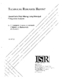## Quantifiable Data Mining Using Principal Component Analysis##### Files
TR_97-25.pdf(1012.81 KB)
Association Rule Mining algorithms operate on a data matrix (e.g., customers x products) to derive rules [2,23]. We propose a single-pass algorithm for mining linear rules in such a matrix based on Principal Component Analysis. PCA detects correlated columns of the matrix, which correspond to, e.g., products that sell together.<P>The first contribution of this work is that we propose to quantify the ﲧoodness of a set of discovered rules. We define the ﲧuessing error : the root-mean-square error of the reconstructed values of the cells of the given matrix, when we pretend that they are unknown. The second contribution is a novel method to guess missing/hidden values from the linear rules that our method derives. For example, if somebody bought $10 of milk and$3 of bread, our rules can ﲧuess the amount spent on, say, butter. Thus, we can perform a variety of important tasks such as forecasting, hat-if' scenarios, outlier detection, and visualization. Moreover, we show that we can compute the principal components with a single pass over the dataset.<P>Experiments on real datasets (e.g., NBA statistics) demonstrate that the proposed method consistently achieves a ﲧuessing error of up to 5 times lower than the straightforward competitor.